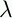September 22, 1999 Perl and the Lambda Calculus Slide #20

# Boolean Value Example

(IF TRUE M N)

(xyz.(x y z)pq.p M N)

(yz.(pq.p y z) M N)

(z.(pq.p M z) N)

(pq.p M N)

(q.M N)

M

Which is what we had hoped for

A similar computation of (IF FALSE M N) yields N.

 NextCopyright © 1999 M-J. Dominus